1619704733

# Classification with Decision trees | Decision Tree Algorithm Explained

We understand Machine Learning, a subset of Artificial Intelligence, as a computer being programmed with the ability to self-learn and improve itself on a particular task. Supervised Learning in Machine Learning allows one to produce or collect data based on previous experience. It helps one to optimize performance criteria using past experience and work on real-time computational problems.

Great Learning brings you this tutorial on Classification using Decision Trees where we understand how classification can be implemented with decision trees using R language. This video discusses the advantages of using tree-based models, followed by looking at a case study to better understand the topic. Then we look at the Gini index, entropy and misclassification error. Following this, we will look at the concept of measuring impurity. Finally, we look at the types of decision tree algorithms! This video teaches Classification using Decision Trees and their key functions and concepts with a variety of demonstrations & examples.

• 0:00:00 Introduction
• 0:01:07 What is Classification?
• 0:03:40 What is Decision Tree Learning?
• 0:15:50 Advantages of using Tree-based Models
• 0:24:46 Gold Loan Case Study
• 1:00:14 Decision Tree in-depth Concept
• 1:16:27 Gini Index, Entropy, Misclassification Error
• 1:31:02 Measuring Impurity
• 1:56:21 Types of Decision Tree algorithms

#machine-learning #artificial-intelligence #developer

## Buddha Community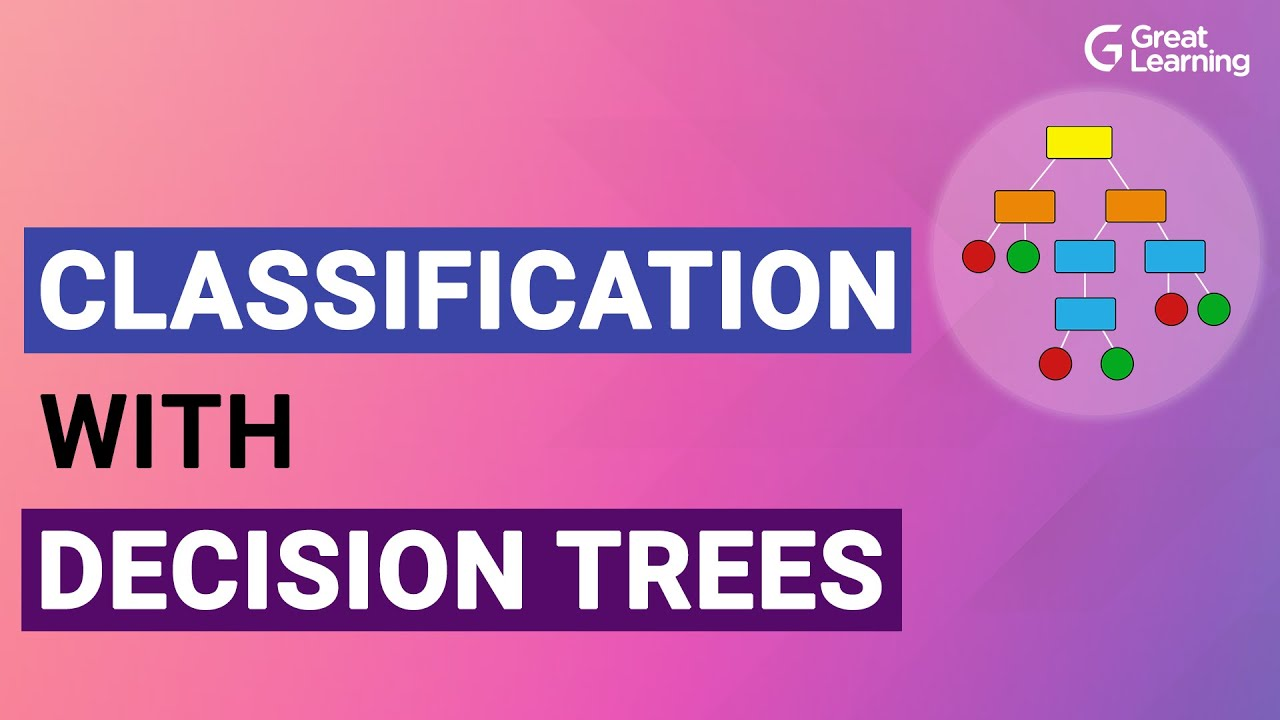1596286260

## Decision Tree -Classification

Decision Tree is one of the most widely used machine learning algorithm. It is a supervised learning algorithm that can perform both classification and regression operations.

As the name suggest, it uses a tree like structure to make decisions on the given dataset. Each internal node of the tree represent a “decision” taken by the model based on any of our attributes. From this decision, we can seperate classes or predict values.

Let’s look at both classification and regression operations one by one.

# Classification

In Classification, each leaf node of our decision tree represents a **class **based on the decisions we make on attributes at internal nodes.

To understand it more properly let us look at an example. I have used the Iris Flower Dataset from sklearn library. You can refer the complete code on Github — Here.

Image for post

A node’s samples attribute counts how many training instances it applies to. For example, 100 training instances have a petal width ≤ 2.45 cm .

A node’s value attribute tells you how many training instances of each class this node applies to. For example, the bottom-right node applies to 0 Iris-Setosa, 0 Iris- Versicolor, and 43 Iris-Virginica.

And a node’s gini attribute measures its impurity: a node is “pure” (gini=0) if all training instances it applies to belong to the same class. For example, since the depth-1 left node applies only to Iris-Setosa training instances, it is pure and its gini score is 0.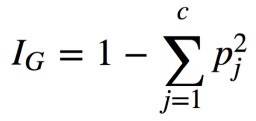Gini Impurity Formula

where, pⱼ is the ratio of instances of class j among all training instances at that node.

Based on the decisions made at each internal node, we can sketch decision boundaries to visualize the model.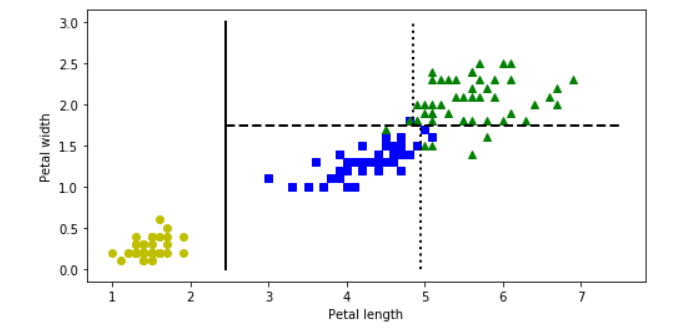But how do we find these boundaries ?

We use Classification And Regression Tree (CART) to find these boundaries.

CART is a simple algorithm that finds an attribute _k _and a threshold _t_ₖat which we get a purest subset. Purest subset means that either of the subsets contain maximum proportion of one particular class. For example, left node at depth-2 has maximum proportion of Iris-Versicolor class i.e 49 of 54. In the _CART cost function, _we split the training set in such a way that we get minimum gini impurity.The CART cost function is given as: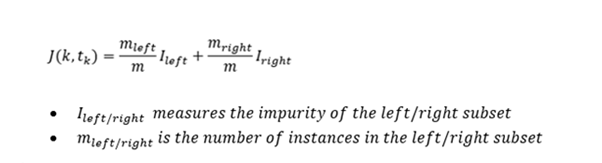After successfully splitting the dataset into two, we repeat the process on either sides of the tree.

We can directly implement Decision tree with the help of Scikit learn library. It has a class called DecisionTreeClassifier which trains the model for us directly and we can adjust the hyperparameters as per our requirements.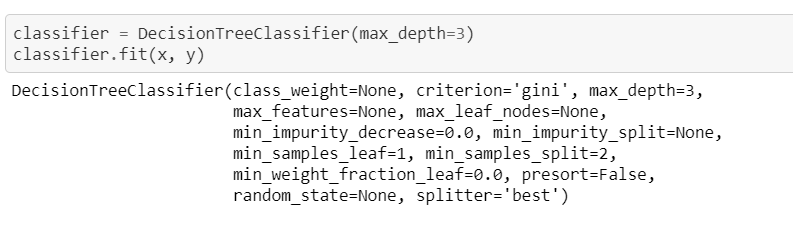#machine-learning #decision-tree #decision-tree-classifier #decision-tree-regressor #deep learning1596428520

## Decision Tree Algorithm..

Decision tree is one of the popular machine learning algorithms which is the stepping stone to understand the ensemble techniques using trees.

Also, Decision Tree algorithm is a hot topic in many of the interviews which are conducted related to data science field.

Understanding Decision Tree…

Decision Tree is more of a kind of Management tool which is used by many professionals to take decisions regarding the resource costs, decision to be made on the basis of filters applied.The best part of a Decision Tree is that it is a non-parametric tool, which means that there are no underlying assumptions about the distribution of the errors or the data. It basically means that the model is constructed based on the observed data.

They are adaptable at solving any kind of problem at hand (classification or regression). Decision Tree algorithms are referred to as CART (Classification and Regression Trees).

Common terms used with Decision trees:

1. Root Node: It represents entire population or sample and this further gets divided into two or more homogeneous sets.
2. Splitting: It is a process of dividing a node into two or more sub-nodes.
3. Decision Node: When a sub-node splits into further sub-nodes, then it is called decision node.
4. Leaf/ Terminal Node: Nodes do not split is called Leaf or Terminal node.
5. **Max_Depth: **The complete journey of a tree from root till the leaf nodes.
6. Branch / Sub-Tree: A sub section of entire tree is called branch or sub-tree.
7. Parent and Child Node: A node, which is divided into sub-nodes is called parent node of sub-nodes whereas sub-nodes are the child of parent node.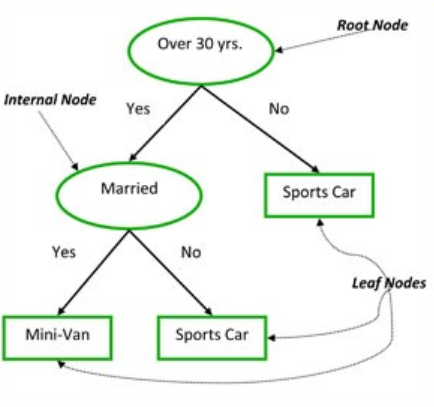classic example to demonstrate a Decision Tree

How a Decision Tree works!

1. First, we will iterate through all possible splits, calculate the purity of each split and pick the split.
2. The purity is compared across all labels and best is chosen. This makes the root node as best predictor.
3. This algorithm is recursive in nature as the groups formed can be sub-divided and the process is repeated till the tree is fully grown.

Main Decision Areas:

1. Determine the best split:

The node with homogeneous class distribution are preferred.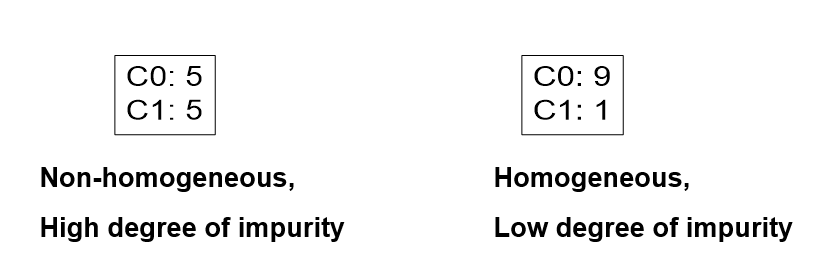2. Measures of Node Impurity: Below are the measures of the impurity

(a). Gini Index

(b). Entropy

Understanding each terminologies with the example:

Let us take a dataset- weather, below is the snapshot of the header of the data: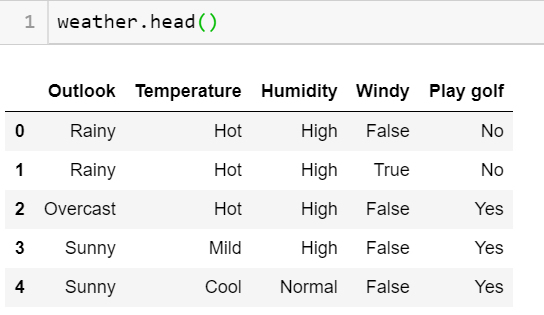Now according to the algorithm written above and the decision points to be considered, we need the feature having maximum information split possible.

Note: At the root node, the impurity level will be maximum with negligible information gain. As we go down the tree, the Entropy reduces with maximizing the Information gain.Therefore, we choose a feature with maximum gain achieved.

#data-science #machine-learning #decision-tree #algorithms #algorithms1596285180

## Decision Trees Classifier

Both of `Regression Trees` and `Classification Trees` are a part of `CART (Classification And Regression Tree) Algorithm`. As we mentioned in Regression Trees article, tree is composed of 3-major parts; root-node, decision-node and terminal/leaf-node.

The criteria used here in node splitting differs from that being used in Regression Trees. As before we will run our example and then learn how the model is being trained.

There are three commonly measures are used in the attribute selection `Gini impurity` measure, is the one used by `CART` classifier. For more information on these, see Wikipedia.

# Data set being used is `iris` data set

``````import numpy as np
import pandas as pd
from sklearn.tree import DecisionTreeClassifier
from sklearn.tree import export_graphviz
from six import StringIO
from IPython.display import Image
# pip/conda install pydotplus
import pydotplus
from sklearn import datasets
xList = iris.data  # Data will be loaded as an array
labels = iris.target
dataset = pd.DataFrame(data=xList,
columns=iris.feature_names)
dataset['target'] = labels
targetNames = iris.target_names
print(targetNames)
print(dataset)
``````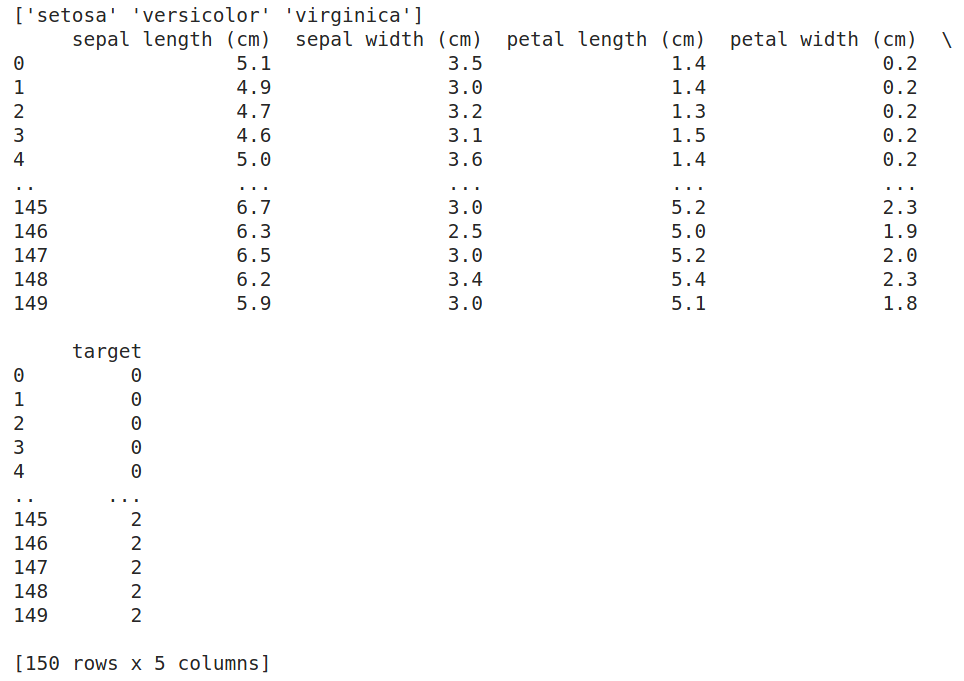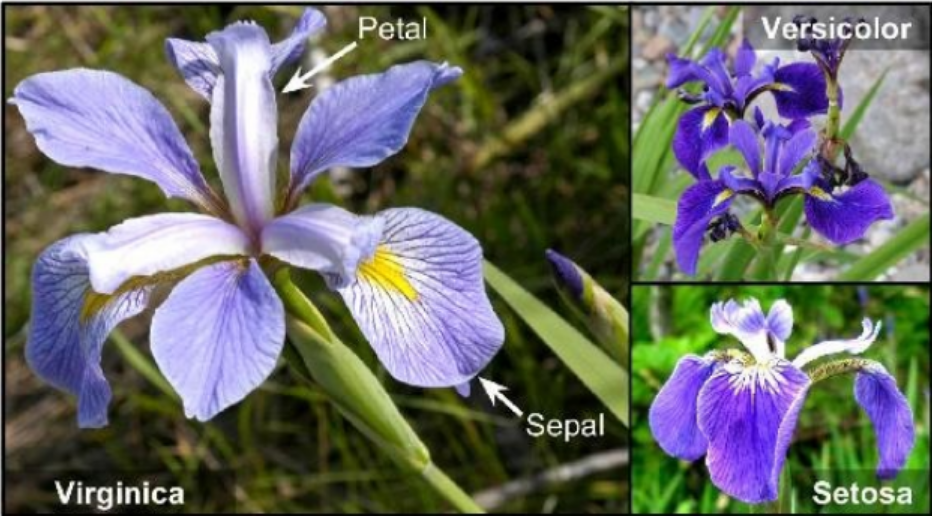Iris Flowers

# How a Binary Decision Tree Generates Predictions

When an observation or row is passed to a non-terminal node, the row answers the node’s question. If it answers yes, the row of attributes is passed to the leaf node below and to the left of the current node. If the row answers no, the row of attributes is passed to the leaf node below and to the right of the current node. The process continues recursively until the row arrives at a terminal (that is, leaf) node where a prediction value is assigned to the row. The value assigned by the leaf node is the mean of the outcomes of the all the training observations that wound up in the leaf node.

# How to split the nodes

Classification trees split a node into two sub-nodes. Splitting into sub-nodes will increase the homogeneity of resultant sub-nodes. In other words, we can say that the purity of the node increases with respect to the target variable. The decision tree splits the nodes on all available variables and then selects the split which results in most homogeneous/pure sub-nodes.

There are major measures being used to determine which attribute/feature is used for splitting and which value within this attribute we will start with. Some of these measures are:

• Gini index (Default in SciKit Learn Classifier).
• Entropy.
• Information gain.

We will start with `Gini index` measure and try to understand it

# Gini Index

Gini index is an impurity measure used to evaluate splits in the dataset. It is calculated by getting the sum of the squared probabilities of each class (target-class) within a certain attribute/feature then benig subtacted from one.

#machine-learning #mls #decision-tree #decision-tree-classifier #classification #deep learning1624442580

## All Machine Learning Algorithms You Should Know in 2021

As my knowledge in machine learning grows, so does the number of machine learning algorithms! This article will cover machine learning algorithms that are commonly used in the data science community.

Keep in mind that I’ll be elaborating on some algorithms more than others simply because this article would be as long as a book if I thoroughly explained every algorithm! I’m also going to try to minimize the amount of math in this article because I know it can be pretty daunting for those who aren’t mathematically savvy. Instead, I’ll try to give a concise summary of each and point out some of the key features.

With that in mind, I’m going to start with some of the more fundamental algorithms and then dive into some newer algorithms like CatBoost, Gradient Boost, and XGBoost.

#### Linear Regression

Linear Regression is one of the most fundamental algorithms used to model relationships between a dependent variable and one or more independent variables. In simpler terms, it involves finding the ‘line of best fit’ that represents two or more variables.

The line of best fit is found by minimizing the squared distances between the points and the line of best fit — this is known as minimizing the sum of squared residuals. A residual is simply equal to the predicted value minus the actual value.

In case it doesn’t make sense yet, consider the image above. Comparing the green line of best fit to the red line, notice how the vertical lines (the residuals) are much bigger for the green line than the red line. This makes sense because the green line is so far away from the points that it isn’t a good representation of the data at all!

If you want to learn more about the math behind linear regression, I would start off with Brilliant’s explanation.

#2021 jan tutorials #overviews #algorithms #decision tree #explained #algorithms1596960480

## Decision Tree (Classification) with sklearn

A classification tree is very alike to a regression tree, besides that it is

used to predict a qualitative response rather than a quantitative one. classification tree, we predict that each observation belongs to the most ordinarily occurring class of training observations in the region to which it belongs.

In the classification, RSS cannot be used for making the binary splits. An alternative to RSS is the classification error rate. we assign an observation in a given region to the most commonly occurring class of training observations in that region,** the classification error rate is simply the fraction of the training observations in that region that do not belong to the most common class:**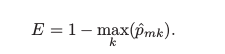p̂mk = proportion of training observations in the mth region that is from the kth class.

classification error is not good enough for tree-growing, and in practice, two other measures are favoured.

1. Gini index is defined by a measure of total variance across the K classes. It takes on a small value if all of the p̂mk ’s are close to 0 or 1. Therefore it is referred to as a **measure of node purity **— a small value shows that a node contains predominantly observations from a single class.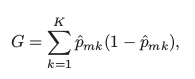2.** Cross-Entropy **is given by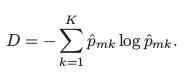Since, 0 ≤ p̂mk ≤ 1, it follows that 0 ≤ −p̂mk log p̂mk. The cross-entropy will take on a value near zero if the p̂mk ’s are all near 0 or near 1. Therefore, the cross-entropy will take on a small value if the mth node is pure. It turns out that the Gini index and the cross-entropy are quite similar numerically.

Two approaches are more sensitive to node purity than is the classification error rate. Any of these three approaches might be used when pruning the tree, but the classification error rate is preferable if the prediction accuracy of the final pruned tree is the goal.

Decision tree classifier using sklearn

To implement the decision tree classifier, we’re going to use scikit-learn, and we’ll import our ‘’DecisionTreeClassifier’’ from sklearn.tree

``````import numpy as np
import pandas as pd
import matplotlib.pyplot as plt
from sklearn import datasets
from sklearn.tree import DecisionTreeClassifier
``````